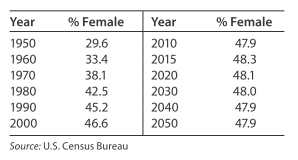# Females in the workforce Suppose the rate of change of the percent p of total U.S. workers who are female can be modeled by d p d t = 2154.18 ( 1.38 t + 64.1 ) 2 percentage points per year, where t is the number of years past 1950. Use integration and the data point for 2040 to find the function p ( t ) that models the percent of the workforce that is female. For selected years from 1950 and projected to 2050, the data in the table show the percent of total U.S. workers who are female. With t as the number of years past 1950, graph these data with the model found in part (a). Comment on the model’s fit to the data.### Mathematical Applications for the ...

11th Edition
Ronald J. Harshbarger + 1 other
Publisher: Cengage Learning
ISBN: 9781305108042

#### Solutions

Chapter
Section### Mathematical Applications for the ...

11th Edition
Ronald J. Harshbarger + 1 other
Publisher: Cengage Learning
ISBN: 9781305108042
Chapter 12.2, Problem 57E
Textbook Problem
1 views

## Females in the workforce Suppose the rate of change of the percent p of total U.S. workers who are female can be modeled by d p d t = 2154.18 ( 1.38 t + 64.1 ) 2 percentage points per year, where t is the number of years past 1950.Use integration and the data point for 2040 to find the function p ( t ) that models the percent of the workforce that is female. For selected years from 1950 and projected to 2050, the data in the table show the percent of total U.S. workers who are female. With t as the number of years past 1950, graph these data with the model found in part (a). Comment on the model’s fit to the data.

This textbook solution is under construction.

### Still sussing out bartleby?

Check out a sample textbook solution.

See a sample solution

#### The Solution to Your Study Problems

Bartleby provides explanations to thousands of textbook problems written by our experts, many with advanced degrees!

Get Started